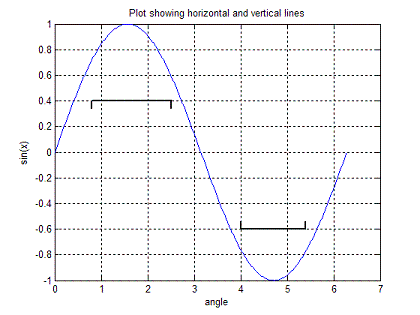# Matlab Plotting - Horizontal Lines and Vertical lines

1.- Basics

2.- Code

3.- Applied Example

4.- Video (alternative code)

### 1.- Basics

We are going to create a simple Matlab function to add horizontal lines (and vertical ones) to any given Matlab-created plot.

For example, let's say that we need to add some indications or annotations to a plot, and we need to display and indicate some upper or lower limits.

The proposed Matlab function will have 5 input parameters:
• Initial value (where the horizontal line will start)
• Final value (where the line will end)
• Y value (vertical position of the line with respect to the plot)
• Direction (to indicate the direction of the annotation: 1 going upwards, or -1 going downwards, like an arrow)
• Vertical range (to display aesthetically proportioned lines)

We plan the usage of our function like this (its name will be 'plot_limit'):

Usage:    L = [L_min L_max y d r];
plot_limit(L);

where
L_min = starting point
L_max = ending point
y = y value of horizontal line
d = direction (1 or -1)
r = y range

So, we need to know in advance where we want to put our line (sure!).

We define our horizontal and vertical values. We arbitrarily define 300 horizontal points and use a linewidth = 1.5. The instruction 'hold on' keeps the current figure, instead of overwriting it. This Matlab code displays just a horizontal line.

a = linspace(L_min, L_max, 300);
b = linspace(y, y, 300);
plot(a,b,'k-','linewidth',1.5)
hold on

Then, we add some tiny vertical lines on each side of the horizontal one. If direction 'd' is 1, the vertical lines are below the horizontal line (going 'up'). If direction 'd' is -1, the vertical lines are above the horizontal one (going 'down'). We take the vertical range into account, in 'r', to display a vertical line of just 3% of the total 'height' of the current figure. You can try different values to suit your needs, obviously. We find the initial or final points of the vertical lines with an 'if-statement'.

### 2.- Code

This is the code to achieve it.

% Initial vertical line
a = linspace(L_min, L_min, 10);
if d == 1
b = linspace(y-r*.03, y, 10);
else
b = linspace(y, y+r*.03, 10);
end
plot(a,b,'k-','linewidth',1.5)
hold on

% Final vertical line
a = linspace(L_max, L_max, 10);
if d == 1
b = linspace(y-r*.03, y, 10);
else
b = linspace(y, y+r*.03, 10);
end
plot(a,b,'k-','linewidth',1.5)

### 3.- Applied Example

Now, we'll test our function with this script.

clear; clc; close all

x = 0 : 2*pi/360 : 2*pi;
y = sin(x);

plot(x,y)
grid on
xlabel('angle')
ylabel('sin(x)')
title('Plot showing horizontal and vertical lines')
hold on
plot_limit([0.8, 2.5, 0.4, 1, 2])
plot_limit([4, 5.4, -0.6, -1, 2])

The result is:The first line starts at 0.8, ends at 2.5, and has a vertical value of 0.4. The vertical lines simulate an up-arrow (d = 1).

The second line starts at 4, ends at 5.4, and has a vertical value of -0.6. Direction goes down (d = -1).

### 4.- Video

From 'Horizontal Lines' to home

From 'Horizontal Lines' to 2D plots